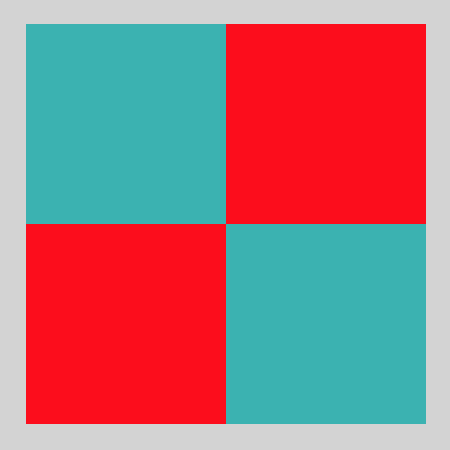# teaching machines

## Multiple Assignment in Twoville

April 12, 2018 by . Filed under public, twoville.

As I dream up animations in Twoville, I find myself generating a lot of shapes that share properties with other shapes. For instance, these two circles have the same radius and color:

a = circle()
b = circle()

a.rgb = [1, 1, 0]
b.rgb = [1, 1, 0]

I really wanted a way to express these repetitive assignments with less code. Something like Ruby’s Array.each method would have worked:

[a, b].each do |shape|
shape.rgb = [1, 1, 0]
end


But even this seems too heavy to me. It introduces a new name—shape—that adds a layer of obfuscation. Then I wondered about setting the properties on the list:

a = circle()
b = circle()

[a, b].rgb = [1, 1, 0]

This syntax makes me want to lick the screen. So concise and clear.

Arrays don’t otherwise support properties, so I was free to hijack their assignment. In the Twoville interpreter, this code effectively expands into the original code above. I haven’t quite figured out if I want the elements of the array to share references to a common right-hand side, or if each should have an independent copy.

I used multiple assignment to plot this checkerboard of squares:And here’s the code behind it:

a = rectangle()
b = rectangle()
c = rectangle()
d = rectangle()

a.position = [100, 200]
b.position = [200, 100]
c.position = [100, 100]
d.position = [200, 200]

[a, b, c, d].size = [100, 100]
[a, b].rgb = [1, 0, 0]
[c, d].rgb = [0.2, 0.7, 0.7]